## Abstract

In this paper, we investigate the Hyers-Ulam stability of the additive-quadratic functional equation $∑ i = 1 n f( x i − 1 n ∑ j = 1 n x j )= ∑ i = 1 n f( x i )−nf( 1 n ∑ i = 1 n x i )$ ($n≥2$) in intuitionistic random normed spaces.

MSC:39B52, 34K36, 46S50, 47S50, 34Fxx.

## 1 Introduction

The concept of stability of a functional equation arises when one replaces a functional equation by an inequality which acts as a perturbation of the equation. The first stability problem concerning group homomorphisms was raised by Ulam  in 1940 and affirmatively solved by Hyers . The result of Hyers was generalized by Aoki  for approximate additive mappings and by Rassias  for approximate linear mappings by allowing the difference Cauchy equation $∥f(x+y)−f(x)−f(y)∥$ to be controlled by $ε( ∥ x ∥ p + ∥ y ∥ p )$. In 1994, a generalization of the Th.M. Rassias’ theorem was obtained by Gǎvruta , who replaced $ε( ∥ x ∥ p + ∥ y ∥ p )$ by a general control function $φ(x,y)$. For more details about the results concerning such problems, the reader is referred to .

The functional equation

$f(x+y)+f(x−y)=2f(x)+2f(y)$
(1.1)

is related to a symmetric bi-additive mapping [17, 18]. It is natural that this equation is called a quadratic functional equation. In particular, every solution of the quadratic equation (1.1) is said to be a quadratic mapping. It is well known that a mapping f between real vector spaces is quadratic if and only if there exists a unique symmetric bi-additive mapping $B 1$ such that $f(x)= B 1 (x,x)$ for all x. The bi-additive mapping $B 1$ is given by $B 1 (x,y)= 1 4 (f(x+y)−f(x−y))$. The Hyers-Ulam stability problem for the quadratic functional equation was solved by Skof . In , Czerwik proved the Hyers-Ulam stability of the function equation (1.1).

Eshaghi Gordji and Khodaei  have established the general solution and investigated the Hyers-Ulam stability for a mixed type of cubic, quadratic and additive functional equation

$f(x+ky)+f(x−ky)= k 2 f(x+y)+ k 2 f(x−y)+2 ( 1 − k 2 ) f(x)$
(1.2)

in quasi-Banach spaces, where k is a nonzero integer with $k≠±1$. Obviously, the function $f(x)=ax+b x 2 +c x 3$ is a solution of the functional equation (1.2). Interesting new results concerning mixed functional equations have recently been obtained by Najati et al. , Jun and Kim [25, 26] as well as for the fuzzy stability of a mixed-type functional equation by Park et al. .

The stability of the mixed functional equation

$∑ i = 1 n f ( x i − 1 n ∑ j = 1 n x j ) = ∑ i = 1 n f( x i )−nf ( 1 n ∑ i = 1 n x i )$
(1.3)

was investigated by Najati and Rassias .

The theory of random normed spaces (RN-spaces) is important as a generalization of the deterministic result of linear normed spaces and also in the study of random operator equations. The RN-spaces may also provide us with the appropriate tools to study the geometry of nuclear physics and have important application in quantum particle physics. The Hyers-Ulam stability of different functional equations in random normed spaces and RN-spaces has been recently studied in Alsina , Eshaghi Gordji et al. [31, 32], Miheţ and Radu , Miheţ, Saadati and Vaezpour [36, 37], and Saadati et al. . Recently, Zhang et al.  investigated the intuitionistic random stability problems for the cubic functional equation.

In this paper, we prove the Hyers-Ulam stability of the additive and quadratic functional equation (1.3) in intuitionistic random spaces.

## 2 Preliminaries

We start our work with the following notion of intuitionistic random normed spaces. In the sequel, we adopt the usual terminology, notations and conventions of the theory of intuitionistic Menger probabilistic normed spaces as in  and .

A measure distribution function is a function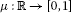, which is left continuous on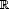, non-decreasing,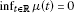and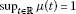.

We denote by D the family of all measure distribution functions, and by H a special element of D defined by

If X is a nonempty set, then $μ:X→D$ is called a probabilistic measure on X and $μ(x)$ is denoted by $μ x$.

A non-measure distribution function is a function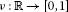, which is right continuous on, non-increasing,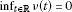and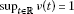.

We denote by B the family of all non-measure distribution functions, and by G a special element of B defined by

If X is a nonempty set, then $ν:X→B$ is called a probabilistic non-measure on X and $ν(x)$ is denoted by $ν x$.

Lemma 2.1 [45, 46]

Consider the set $L ∗$ and the operation $≤ L ∗$ defined by

$L ∗ = { ( x 1 , x 2 ) ∈ [ 0 , 1 ] × [ 0 , 1 ] : x 1 + x 2 ≤ 1 } , ∀ ( x 1 , x 2 ) , ( y 1 , y 2 ) ∈ L ∗ , ( x 1 , x 2 ) ≤ L ∗ ( y 1 , y 2 ) ⟺ x 1 ≤ y 1 , x 2 ≥ y 2 .$

Then $( L ∗ , ≤ L ∗ )$ is a complete lattice.

We denote the units by $0 L ∗ =(0,1)$ and $1 L ∗ =(1,0)$. Classically, for all $x∈[0,1]$, a triangular norm $∗=T$ on [0,1] is defined as an increasing, commutative, associative mapping $T: [ 0 , 1 ] 2 ⟶[0,1]$ satisfying $T(1,x)=1∗x=x$, and a triangular conorm $S=⋄$ is defined as an increasing, commutative, associative mapping $S: [ 0 , 1 ] 2 →[0,1]$ satisfying $S(0,x)=0⋄x=x$.

By use of the lattice $( L ∗ , ≤ L ∗ )$, these definitions can be straightforwardly extended.

Definition 2.2 

A triangular norm (t-norm) on $L ∗$ is a mapping $ϒ: ( L ∗ ) 2 ⟶ L ∗$ satisfying the following conditions:

1. (i)

$∀x∈ L ∗$, $ϒ(x, 1 L ∗ )=x$ (boundary condition);

2. (ii)

$∀(x,y)∈ ( L ∗ ) 2$, $ϒ(x,y)=ϒ(y,x)$ (commutativity);

3. (iii)

$∀(x,y,z)∈ ( L ∗ ) 3$, $ϒ(x,ϒ(y,z))=ϒ(ϒ(x,y),z)$ (associativity);

4. (iv)

$∀(x, x ′ ,y, y ′ )∈ ( L ∗ ) 4$, $x ≤ L ∗ x ′$, $y ≤ L ∗ y ′ ⟹ϒ(x,y) ≤ L ∗ ϒ( x ′ , y ′ )$ (monotonicity).

If $( L ∗ , ≤ L ∗ ,ϒ)$ is an Abelian topological monoid with unit $1 L ∗$, then ϒ is said to be a continuous t-norm.

Definition 2.3 

A continuous t-norm ϒ on $L ∗$ is said to be continuous t-representable if there exist a continuous t-norm and a continuous t-conorm on [0,1] such that

$∀x=( x 1 , x 2 ),y=( y 1 , y 2 )∈ L ∗ ,ϒ(x,y)=( x 1 ∗ y 1 , x 2 ⋄ y 2 ).$

Typical examples of continuous t-representable are $ϒ(a,b)=( a 1 b 1 ,min{ a 2 + b 2 ,1})$ and $M(a,b)=(min{ a 1 , b 1 },max{ a 2 , b 2 })$ for all $a=( a 1 , a 2 )$, $b=( b 1 , b 2 )∈ L ∗$.

Now, we define a sequence $ϒ n$ recursively by $ϒ 1 =ϒ$ as

$ϒ n ( x ( 1 ) , x ( 2 ) , … , x ( n + 1 ) ) =ϒ ( ϒ n − 1 ( x ( 1 ) , x ( 2 ) , … , x ( n ) ) , x ( n + 1 ) )$

for all $x ( 1 ) ,…, x ( n + 1 ) ∈ L ∗$ and $n≥2$.

Recall that if ϒ is a t-norm and ${ x ( n ) }$ is a given sequence of numbers in $L ∗$, $ϒ i = 1 n x ( i )$ is defined recurrently by

for all $x ( i ) ∈ L ∗$. $ϒ i = n ∞ x ( i )$ is defined as $ϒ i = 1 ∞ x ( n + i )$.

A negator on $L ∗$ is any decreasing mapping $ℵ: L ∗ ⟶ L ∗$ satisfying $ℵ( 0 L ∗ )=( 1 L ∗ )$ and $ℵ( 1 L ∗ )=( 0 L ∗ )$. If $ℵℵ(x)=x$ for all $x∈ L ∗$, then is called an involutive negator. A negator on [0,1] is a decreasing mapping $N:[0,1]⟶[0,1]$ satisfying $N(0)=1$ and $N(1)=0$. $N s$ denotes the standard negator on $[0,1]$ defined by $N s (x)=1−x$ for all $x∈[0,1]$.

Definition 2.4 . Let μ and ν be measure and non-measure distribution functions from $X×(0,+∞)$ to $[0,1]$ such that $μ x (t)+ ν x (t)≤1$ for all $x∈X$ and all $t>0$. The triple $(X, Λ μ , ν ,ϒ)$ is said to be an intuitionistic random normed space (briefly IRN-space) if X is a vector space, ϒ is a continuous t-representable, and $Λ μ , ν :X×(0,+∞)→ L ∗$ is a mapping such that the following conditions hold for all $x,y∈X$ and all $t,s≥0$:

($IR N 1$) $Λ μ , ν (x,0)= 0 L ∗$;

($IR N 2$) $Λ μ , ν (x,t)= 1 L ∗$ if and only if $x=0$;

($IR N 3$) $Λ μ , ν (αx,t)= Λ μ , ν (x, t | α | )$ for all $α≠0$;

($IR N 4$) $Λ μ , ν (x+y,t+s) ≥ L ∗ ϒ( Λ μ , ν (x,t), Λ μ , ν (y,s))$.

In this case, $Λ μ , ν$ is called an intuitionistic random norm. Here, $Λ μ , ν (x,t)=( μ x (t), ν x (t))$.

Every normed space $(X,∥⋅∥)$ defines an IRN-space $(X, Λ μ , ν ,ϒ)$, where $Λ μ , ν (x,t)=( t t + ∥ x ∥ , ∥ x ∥ t + ∥ x ∥ )$ for all $t>0$ and $ϒ(a,b)=( a 1 b 1 ,min{ a 2 + b 2 ,1})$ for all $a=( a 1 , a 2 )$, $b=( b 1 , b 2 )∈ L ∗$. This space is called the induced IRN-space.

Definition 2.5 Let $(X, Λ μ , ν ,ϒ)$ be an IRN-space.

1. (1)

A sequence ${ x n }$ in X is said to be convergent to x in X if, $Λ μ , ν ( x n −x,t)→ 1 L ∗$ as $n→∞$ for every $t>0$.

2. (2)

A sequence ${ x n }$ in X is called Cauchy if, for every $ϵ>0$ and $t>0$, there exists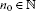such that $Λ μ , ν ( x n − x m ,t) ≥ L ∗ ( N s (ε),ε)$ for every $m,n≥ n 0$, where $N s$ is a standard negator.

3. (3)

An IRN-space $(X, Λ μ , ν ,ϒ)$ is said to be complete if and only if every Cauchy sequence in X is convergent to a point in X.

From now on, let X be a linear space and $(Y, Λ μ , ν ,ϒ)$ be a complete IRN-space.

For convenience, we use the following abbreviation for a given mapping $f:X→Y$:

$Δf( x 1 ,…, x n )= ∑ i = 1 n f ( x i − 1 n ∑ j = 1 n x j ) − ∑ i = 1 n f( x i )+nf ( 1 n ∑ i = 1 n x i )$

for all $x 1 ,…, x n ∈X$, where $n≥2$ is a fixed integer.

## 3 Results in intuitionistic random spaces

In the following theorem, we prove the Hyers-Ulam stability of the functional equation (1.3) in IRN-spaces for quadratic mappings.

Theorem 3.1 Let $ξ,ζ: X n → D +$ ($ξ( x 1 ,…, x n )$ is denoted by $ξ x 1 , … , x n$, $ζ( x 1 ,…, x n )$ is denoted by $ζ x 1 , … , x n$ and $( ξ x 1 , … , x n (t), ζ x 1 , … , x n (t))$ is denoted by $Φ ξ , ζ ( x 1 ,…, x n ,t)$) be mappings such that

$lim m → ∞ Φ ξ , ζ ( 2 m x 1 , … , 2 m x n , 2 2 m t ) = 1 L ∗$
(3.1)

for all $x 1 ,…, x n ∈X$ and all $t>0$, and

$lim m → ∞ ϒ i = 1 ∞ ( M μ , ν e ( 2 m + i − 1 x , 2 2 m + i t ) ) = 1 L ∗$
(3.2)

for all $x∈X$ and all $t>0$. Suppose that an even mapping $f:X→Y$ with $f(0)=0$ satisfies the inequality

$Λ μ , ν ( Δ f ( x 1 , … , x n ) , t ) ≥ L ∗ Φ ξ , ζ ( x 1 ,…, x n ,t)$
(3.3)

for all $x 1 ,…, x n ∈X$ and all $t>0$. Then there exists a unique quadratic mapping $Q:X→Y$ such that

$Λ μ , ν ( f ( x ) − Q ( x ) , t ) ≥ L ∗ ϒ i = 1 ∞ ( M μ , ν e ( 2 i − 1 x , 2 i t ) )$
(3.4)

for all $x∈X$ and all $t>0$, where

$M μ , ν e ( x , t ) = ϒ ( Φ ξ , ζ ( n x , n x , 0 , … , 0 , ( n − 1 ) t ) , ϒ ( ϒ ( Φ ξ , ζ ( n x , 0 , … , 0 , n − 1 8 t ) , ϒ ( Φ ξ , ζ ( 0 , n x , … , n x , n − 1 16 t ) , ϒ ( Φ ξ , ζ ( n x , 0 , … , 0 , n − 1 16 t ) , Φ ξ , ζ ( n x , 0 , … , 0 , n − 1 8 n t ) ) ) ) , ϒ ( Φ ξ , ζ ( x , ( n − 1 ) x , 0 , … , 0 , n − 1 8 t ) , ϒ ( Φ ξ , ζ ( 0 , n x , … , n x , n − 1 16 t ) , ϒ ( Φ ξ , ζ ( n x , 0 , … , 0 , ( n − 1 ) 16 t ) , Φ ξ , ζ ( n x , 0 , … , 0 , n − 1 8 n t ) ) ) ) ) ) .$
(3.5)

Proof Letting $x 1 =n x 1$ and $x i =n x 2$ ($i=2,…,n$) in (3.3) and using the evenness of f, we get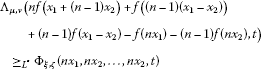(3.6)

for all $x 1 , x 2 ∈X$ and all $t>0$. Interchanging $x 1$ with $x 2$ in (3.6) and using the evenness of f, we get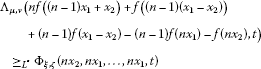(3.7)

for all $x 1 , x 2 ∈X$ and all $t>0$. It follows from (3.6) and (3.7) that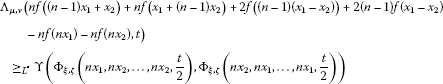(3.8)

for all $x 1 , x 2 ∈X$ and all $t>0$. Setting $x 1 =n x 1$, $x 2 =−n x 2$ and $x i =0$ ($i=3,…,n$) in (3.3) and using the evenness of f, we get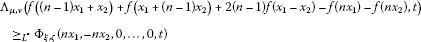(3.9)

for all $x 1 , x 2 ∈X$ and all $t>0$. So it follows from (3.8) and (3.9) that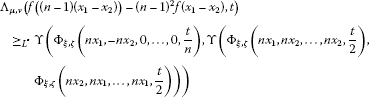(3.10)

for all $x 1 , x 2 ∈X$ and all $t>0$. So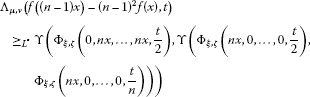(3.11)

for all $x∈X$ and all $t>0$. Putting $x 1 =nx$ and $x i =0$ ($i=2,…,n$) in (3.3), we obtain

$Λ μ , ν ( f ( n x ) − f ( ( n − 1 ) x ) − ( 2 n − 1 ) f ( x ) , t ) ≥ L ∗ Φ ξ , ζ (nx,0,…,0,t)$
(3.12)

for all $x∈X$ and all $t>0$. It follows from (3.11) and (3.12) that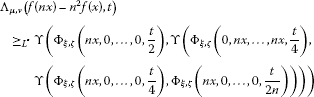(3.13)

for all $x∈X$ and all $t>0$. Letting $x 2 =−(n−1) x 1$ in (3.9) and replacing $x 1$ by $x n$ in the obtained inequality, we get

$Λ μ , ν ( f ( ( n − 1 ) x ) − f ( ( n − 2 ) x ) − ( 2 n − 3 ) f ( x ) , t ) ≥ L ∗ Φ ξ , ζ ( x , ( n − 1 ) x , 0 , … , 0 , t )$
(3.14)

for all $x∈X$ and all $t>0$. It follows from (3.11) and (3.14) that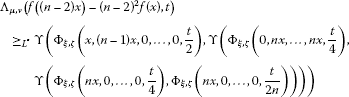(3.15)

for all $x∈X$ and all $t>0$. Applying (3.13) and (3.15), we get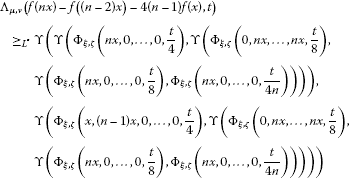(3.16)

for all $x∈X$ and all $t>0$. Setting $x 1 = x 2 =nx$ and $x i =0$ ($i=3,…,n$) in (3.3), we obtain

$Λ μ , ν ( f ( ( n − 2 ) x ) + ( n − 1 ) f ( 2 x ) − f ( n x ) , t ) ≥ L ∗ Φ ξ , ζ (nx,nx,0,…,0,2t)$
(3.17)

for all $x∈X$ and all $t>0$. It follows from (3.16) and (3.17) that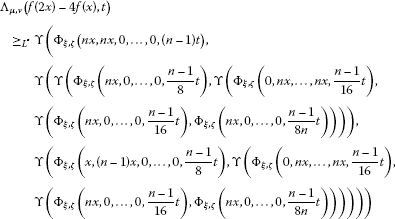for all $x∈X$ and all $t>0$. It follows from (3.5) that

$Λ μ , ν ( f ( 2 x ) 2 2 − f ( x ) , t ) ≥ L ∗ M μ , ν e ( x , 2 2 t ) ≥ L ∗ M μ , ν e (x,2t)$
(3.18)

for all $x∈X$ and all $t>0$, which implies that

$Λ μ , ν ( f ( 2 k + 1 x ) 2 2 ( k + 1 ) − f ( 2 k x ) 2 2 k , t ) ≥ L ∗ M μ , ν e ( 2 k x , 2 2 ( k + 1 ) t )$
(3.19)

for all $x∈X$, all $t>0$ and all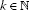. It follows from (3.19) and ($IR N 4$) that

$Λ μ , ν ( f ( 2 2 x ) 2 4 − f ( x ) , t ) ≥ L ∗ ϒ ( Λ μ , ν ( f ( 2 2 x ) 2 4 − f ( 2 x ) 2 2 , t 2 ) , Λ μ , ν ( f ( 2 x ) 2 2 − f ( x ) , t 2 ) ) ≥ L ∗ ϒ ( M μ , ν e ( 2 x , 2 3 t ) , M μ , ν e ( x , 2 t ) ) ≥ L ∗ ϒ ( M μ , ν e ( 2 x , 2 2 t ) , M μ , ν e ( x , 2 t ) )$

and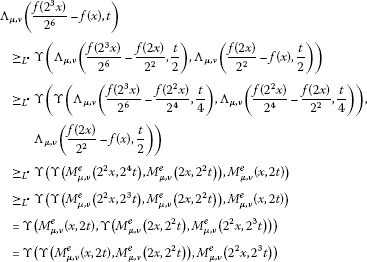for all $x∈X$ and all $t>0$. Thus

$Λ μ , ν ( f ( 2 m x ) 2 2 m − f ( x ) , t ) ≥ L ∗ ϒ i = 1 m ( M μ , ν e ( 2 i − 1 x , 2 i t ) )$
(3.20)

for all $x∈X$ and all $t>0$. In order to prove the convergence of the sequence ${ f ( 2 m x ) 2 2 m }$, we replace x with $2 m ′ x$ in (3.20) to find that

$Λ μ , ν ( f ( 2 m + m ′ x ) 2 2 ( m + m ′ ) − f ( 2 m ′ x ) 2 2 m ′ , t ) ≥ L ∗ ϒ i = 1 m ( M μ , ν e ( 2 m ′ + i − 1 x , 2 2 m ′ + i t ) )$
(3.21)

for all $x∈X$ and all $t>0$. Since the right-hand side of the inequality (3.21) tends to $1 L ∗$ as $m ′$ and m tend to infinity, the sequence ${ f ( 2 m x ) 2 2 m }$ is a Cauchy sequence. Therefore, one can define the mapping $Q:X→Y$ by $Q(x):= lim m → ∞ 1 2 2 m f( 2 m x)$ for all $x∈X$. Now, if we replace $x 1 ,…, x n$ with $2 m x 1 ,…, 2 m x n$ in (3.3) respectively, then

$Λ μ , ν ( Δ f ( 2 m x 1 , … , 2 m x n ) 2 2 m , t ) ≥ L ∗ Φ ξ , ζ ( 2 m x 1 , … , 2 m x n , 2 2 m t )$
(3.22)

for all $x 1 ,…, x n ∈X$ and all $t>0$. By letting $m→∞$ in (3.22), we find that $Λ μ , ν (ΔQ( x 1 ,…, x n ),t)= 1 L ∗$ for all $t>0$, which implies $ΔQ( x 1 ,…, x n )=0$. Thus Q satisfies (1.3). Hence the mapping $Q:X→Y$ is quadratic.

To prove (3.4), take the limit as $m→∞$ in (3.20).

Finally, to prove the uniqueness of the quadratic mapping Q subject to (3.4), let us assume that there exists a quadratic mapping $Q ′$ which satisfies (3.4). Since $Q( 2 m x)= 2 2 m Q(x)$ and $Q ′ ( 2 m x)= 2 2 m Q ′ (x)$ for all $x∈X$ and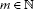, from (3.4) and (3.2) it follows that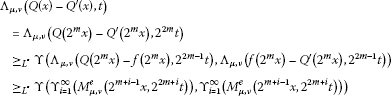(3.23)

for all $x∈X$ and all $t>0$. By letting $m→∞$ in (3.23), we find that $Q= Q ′$. □

Corollary 3.2 Let $(X, Λ μ ′ , ν ′ ,ϒ)$ be an IRN-space and let $(Y, Λ μ , ν ,ϒ)$ be a complete IRN-space. If $f:X→Y$ is a mapping such that

$Λ μ , ν ( Δ f ( x 1 , … , x n ) , t ) ≥ L ∗ Λ μ ′ , ν ′ ( x 1 +⋯+ x n ,t)$

for all $x 1 ,…, x n ∈X$ and all $t>0$, and

$lim m → ∞ ϒ i = 1 ∞ ( M μ ′ , ν ′ e ( 2 m + i − 1 x , 2 2 m + i t ) ) = 1 L ∗$

for all $x∈X$ and all $t>0$, then there exists a unique quadratic mapping $Q:X→Y$ such that

$Λ μ , ν ( f ( x ) − Q ( x ) , t ) ≥ L ∗ ϒ i = 1 ∞ ( M μ ′ , ν ′ e ( 2 i − 1 x , 2 i t ) )$

for all $x∈X$ and all $t>0$, where

$M μ ′ , ν ′ e ( x , t ) = ϒ ( Λ μ ′ , ν ′ ( x , n − 1 2 n t ) , ϒ ( ϒ ( Λ μ ′ , ν ′ ( x , n − 1 8 n t ) , ϒ ( Λ μ ′ , ν ′ ( x , 1 16 n t ) , ϒ ( Λ μ ′ , ν ′ ( x , n − 1 16 n t ) , Λ μ ′ , ν ′ ( x , n − 1 8 n 2 t ) ) ) ) , ϒ ( Λ μ ′ , ν ′ ( x , n − 1 8 n t ) , ϒ ( Λ μ ′ , ν ′ ( x , 1 16 n t ) , ϒ ( Λ μ ′ , ν ′ ( x , ( n − 1 ) 16 n t ) , Λ μ ′ , ν ′ ( x , n − 1 8 n 2 t ) ) ) ) ) ) .$

Proof Let $Φ ξ , ζ ( x 1 ,…, x n ,t)= Λ μ ′ , ν ′ ( x 1 +⋯+ x n ,t)$. Then the corollary follows immediately from Theorem 3.1. □

Now, we prove the Hyers-Ulam stability of the functional equation (1.3) in IRN-spaces for additive mappings.

Theorem 3.3 Let $ξ,ζ: X n → D +$ be mappings such that

$lim m → ∞ Φ ξ , ζ ( 2 m x 1 , … , 2 m x n , 2 m t ) = 1 L ∗$
(3.24)

for all $x 1 ,…, x n ∈X$ and all $t>0$, and

$lim m → ∞ ϒ i = 1 ∞ ( M μ , ν o ( 2 m + i − 1 x , 2 m − 1 t ) ) = 1 L ∗$
(3.25)

for all $x∈X$ and all $t>0$. Suppose that an odd mapping $f:X→Y$ satisfies (3.3) for all $x 1 ,…, x n ∈X$ and all $t>0$. Then there exists a unique additive mapping $A:X→Y$ such that

$Λ μ , ν ( f ( x ) − A ( x ) , t ) ≥ L ∗ ϒ i = 1 ∞ ( M μ , ν o ( 2 i − 1 x , t ) )$
(3.26)

for all $x∈X$ and all $t>0$, where

$M μ , ν o ( x , t ) = ϒ ( Φ ξ , ζ ( 2 x , 0 , … , 0 , t 2 ) , ϒ ( Φ ξ , ζ ( x , x , 0 , … , 0 , t 2 n ) , ϒ ( Φ ξ , ζ ( x , − x , … , − x , t 4 ) , Φ ξ , ζ ( − x , x , … , x , t 4 ) ) ) ) .$

Proof Letting $x 1 =n x 1$ and $x i =n x 1 ′$ ($i=2,…,n$) in (3.3) and using the oddness of f, we get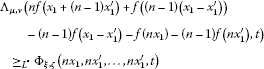(3.27)

for all $x 1 , x 1 ′ ∈X$ and all $t>0$. Interchanging $x 1$ with $x 1 ′$ in (3.27) and using the oddness of f, we get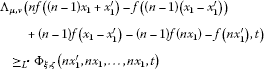(3.28)

for all $x 1 , x 1 ′ ∈X$ and all $t>0$. It follows from (3.27) and (3.28) that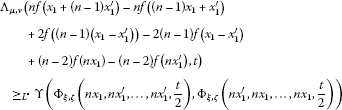(3.29)

for all $x 1 , x 1 ′ ∈X$ and all $t>0$. Setting $x 1 =n x 1$, $x 2 =−n x 1 ′$ and $x i =0$ ($i=3,…,n$) in (3.3) and using the oddness of f, we get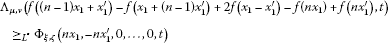(3.30)

for all $x 1 , x 1 ′ ∈X$ and all $t>0$. It follows from (3.29) and (3.30) that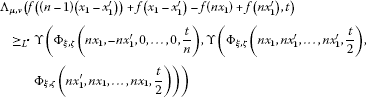(3.31)

for all $x 1 , x 1 ′ ∈X$ and all $t>0$. Putting $x 1 =n( x 1 − x 1 ′ )$ and $x i =0$ ($i=2,…,n$) in (3.3), we obtain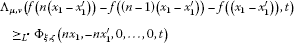(3.32)

for all $x 1 , x 1 ′ ∈X$ and all $t>0$. It follows from (3.31) and (3.32) that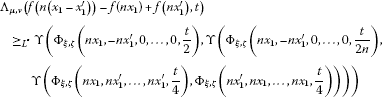(3.33)

for all $x 1 , x 1 ′ ∈X$ and all $t>0$. Replacing $x 1$ and $x 1 ′$ by $x n$ and $− x n$ in (3.33), respectively, we obtain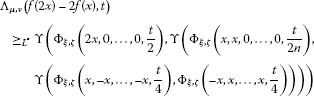for all $x∈X$ and all $t>0$. Therefore,

$Λ μ , ν ( f ( 2 x ) 2 − f ( x ) , t ) ≥ L ∗ M μ , ν o (x,2t) ≥ L ∗ M μ , ν o (x,t)$
(3.34)

for all $x∈X$ and all $t>0$, which implies that

$Λ μ , ν ( f ( 2 k + 1 x ) 2 k + 1 − f ( 2 k x ) 2 k , t ) ≥ L ∗ M μ , ν o ( 2 k x , 2 k + 1 t )$
(3.35)

for all $x∈X$, $t>0$ and. It follows from (3.35) and ($IR N 4$) that

$Λ μ , ν ( f ( 2 2 x ) 2 2 − f ( x ) , t ) ≥ L ∗ ϒ ( Λ μ , ν ( f ( 2 2 x ) 2 2 − f ( 2 x ) 2 , t 2 ) , Λ μ , ν ( f ( 2 x ) 2 − f ( x ) , t 2 ) ) ≥ L ∗ ϒ ( M μ , ν o ( 2 x , 2 t ) , M μ , ν 0 ( x , t ) ) ≥ L ∗ ϒ ( M μ , ν o ( 2 x , t ) , M μ , ν o ( x , t ) )$

and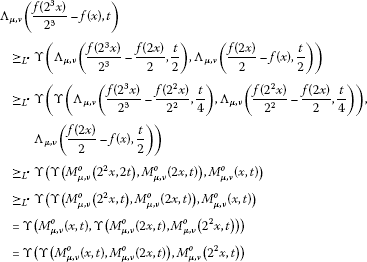for all $x∈X$ and all $t>0$. Thus

$Λ μ , ν ( f ( 2 m x ) 2 m − f ( x ) , t ) ≥ L ∗ ϒ i = 1 m ( M μ , ν o ( 2 i − 1 x , t ) )$
(3.36)

for all $x∈X$ and all $t>0$. In order to prove the convergence of the sequence ${ f ( 2 m x ) 2 m }$, we replace x with $2 m ′ x$ in (3.36) to find that

$Λ μ , ν ( f ( 2 m + m ′ x ) 2 m + m ′ − f ( 2 m ′ x ) 2 m ′ , t ) ≥ L ∗ ϒ i = 1 m ( M μ , ν o ( 2 m ′ + i − 1 x , 2 m ′ t ) )$
(3.37)

for all $x∈X$ and all $t>0$. Since the right-hand side of the inequality (3.37) tends to $1 L ∗$ as $m ′$ and m tend to infinity, the sequence ${ f ( 2 m x ) 2 m }$ is a Cauchy sequence. Therefore, one can define the mapping $A:X→Y$ by $A(x):= lim m → ∞ 1 2 m f( 2 m x)$ for all $x∈X$. Now, if we replace $x 1 ,…, x n$ with $2 m x 1 ,…, 2 m x n$ in (3.3) respectively, then

$Λ μ , ν ( Δ f ( 2 m x 1 , … , 2 m x n ) 2 m , t ) ≥ L ∗ Φ ξ , ζ ( 2 m x 1 , … , 2 m x n , 2 m t )$
(3.38)

for all $x 1 ,…, x n ∈X$ and all $t>0$. By letting $m→∞$ in (3.38), we find that $Λ μ , ν (ΔA( x 1 ,…, x n ),t)= 1 L ∗$ for all $t>0$, which implies $ΔA( x 1 ,…, x n )=0$. Thus A satisfies (1.3). Hence the mapping $A:X→Y$ is additive. To prove (3.26), take the limit as $m→∞$ in (3.36).

The rest of the proof is similar to the proof of Theorem 3.1. □

Corollary 3.4 Let $(X, Λ μ ′ , ν ′ ,ϒ)$ be an IRN-space and let $(Y, Λ μ , ν ,ϒ)$ be a complete IRN-space. If $f:X→Y$ is a mapping such that

$Λ μ , ν ( Δ f ( x 1 , … , x n ) , t ) ≥ L ∗ Λ μ ′ , ν ′ ( x 1 +⋯+ x n ,t)$

for all $x 1 ,…, x n ∈X$ and all $t>0$, and

$lim m → ∞ ϒ i = 1 ∞ ( M μ ′ , ν ′ o ( 2 m + i − 1 x , 2 m − 1 t ) ) = 1 L ∗$

for all $x∈X$ and all $t>0$, then there exists a unique additive mapping $A:X→Y$ such that

$Λ μ , ν ( f ( x ) − A ( x ) , t ) ≥ L ∗ ϒ i = 1 ∞ ( M μ ′ , ν ′ o ( 2 i − 1 x , t ) )$

for all $x∈X$ and all $t>0$, where

$M μ ′ , ν ′ o ( x , t ) = ϒ ( Λ μ ′ , ν ′ ( x , t 4 ) , ϒ ( Λ μ ′ , ν ′ ( x , t 4 n ) , ϒ ( Λ μ ′ , ν ′ ( x , t 4 ( 2 − n ) ) , Λ μ ′ , ν ′ ( x , t 4 ( n − 2 ) ) ) ) ) .$

The main result of this paper is the following:

Theorem 3.5 Let $ξ,ζ: X n → D +$ be mappings satisfying (3.24) and (3.25) for all $x 1 ,…, x n ,x∈X$ and all $t>0$. Suppose that a mapping $f:X→Y$ with $f(0)=0$ satisfies (3.3) for all $x 1 ,…, x n ∈X$ and all $t>0$. Then there exist an additive mapping $A:X→Y$ and a quadratic mapping $Q:X→Y$ satisfying (1.3) and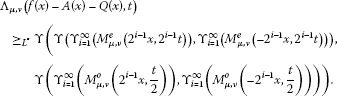(3.39)

Proof Let $f e (x)= 1 2 (f(x)+f(−x))$ for all $x∈X$. Then $f e (0)=0$, $f e (−x)= f e (x)$ and

$Λ μ , ν ( Δ f e ( x 1 , … , x n ) , t ) = Λ μ , ν ( Δ f ( x 1 , … , x n ) + Δ f ( − x 1 , … , − x n ) 2 , t ) ≥ L ∗ ϒ ( Λ μ , ν ( Δ f ( x 1 , … , x n ) , t ) , Λ μ , ν ( Δ f ( − x 1 , … , − x n ) , t ) ) ≥ L ∗ ϒ ( Φ ξ , ζ ( x 1 , … , x n , t ) , Φ ξ , ζ ( − x 1 , … , − x n , t ) )$

for all $x 1 ,…, x n ∈X$ and all $t>0$. By Theorem 3.1, there exists a quadratic mapping $Q:X→Y$ such that

$Λ μ , ν ( f e ( x ) − Q ( x ) , t ) ≥ L ∗ ϒ ( ϒ i = 1 ∞ ( M μ , ν e ( 2 i − 1 x , 2 i t ) ) , ϒ i = 1 ∞ ( M μ , ν e ( − 2 i − 1 x , 2 i t ) ) )$
(3.40)

for all $x∈X$ and all $t>0$.

On the other hand, let $f o (x)= 1 2 (f(x)−f(−x))$ for all $x∈X$. Then $f o (0)=0$, $f o (−x)=− f o (x)$. By Theorem 3.3, there exists an additive mapping $A:X→Y$ such that

$Λ μ , ν ( f o ( x ) − A ( x ) , t ) ≥ L ∗ ϒ ( ϒ i = 1 ∞ ( M μ , ν o ( 2 i − 1 x , t ) ) , ϒ i = 1 ∞ ( M μ , ν o ( − 2 i − 1 x , t ) ) )$
(3.41)

for all $x∈X$ and all $t>0$. Hence (3.39) follows from (3.40) and (3.41). □

Corollary 3.6 Let $(X, Λ μ ′ , ν ′ ,ϒ)$ be an IRN-space and let $(Y, Λ μ , ν ,ϒ)$ be a complete IRN-space. If $f:X→Y$ is a mapping such that

$Λ μ , ν ( Δ f ( x 1 , … , x n ) , t ) ≥ L ∗ Λ μ ′ , ν ′ ( x 1 +⋯+ x n ,t)$

for all $x 1 ,…, x n ∈X$ and all $t>0$, and

$lim m → ∞ ϒ i = 1 ∞ ( M μ ′ , ν ′ o ( 2 m + i − 1 x , 2 m − 1 t ) ) = 1 L ∗$

for all $x∈X$ and all $t>0$, then there exist an additive mapping $A:X→Y$ and a quadratic mapping $Q:X→Y$ such that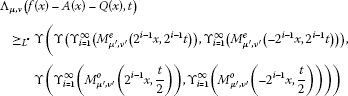for all $x∈X$ and all $t>0$.

Now, we give an example to validate the result of quadratic mappings as follows:

Example Let $(X,∥⋅∥)$ be a Banach space, $(X, Λ μ , ν ,M)$ an IRN-space in which

$Λ μ , ν (x,t)= ( t t + ∥ x ∥ , ∥ x ∥ t + ∥ x ∥ )$

and let $(Y, Λ μ , ν ,M)$ be a complete IRN-space for all $x∈X$. Define a mapping $f:X→Y$ by $f(x)= x 2 + x 0$, where $x 0$ is a unit vector in X. A straightforward computation shows that, for all $t>0$,

$Λ μ , ν ( Δ f ( x 1 , … , x n ) , t ) ≥ L ∗ Λ μ , ν ( x 1 +⋯+ x n ,t)$

and

$lim m → ∞ M i = 1 ∞ ( M μ , ν e ( 2 m + i − 1 x , 2 2 m + i t ) ) = lim m → ∞ lim k → ∞ M i = 1 k ( M μ , ν e ( x , 2 m + 1 t ) ) = lim m → ∞ ( M μ , ν e ( x , 2 m + 1 t ) ) = 1 L ∗ .$

Therefore, all the conditions of Theorem 3.1 hold, and so there exists a unique quadratic mapping $Q:X→Y$ such that $Λ μ , ν (f(x)−Q(x),t) ≥ L ∗ M μ , ν e (x,2t)$.

## References

1. 1.

Ulam SM: A Collection of the Mathematical Problems. Interscience, New York; 1960.

2. 2.

Hyers DH: On the stability of the linear functional equation. Proc. Natl. Acad. Sci. USA 1941, 27: 222–224. 10.1073/pnas.27.4.222

3. 3.

Aoki T: On the stability of the linear transformation in Banach spaces. J. Math. Soc. Jpn. 1950, 2: 64–66. 10.2969/jmsj/00210064

4. 4.

Rassias TM: On the stability of the linear mapping in Banach spaces. Proc. Am. Math. Soc. 1978, 72: 297–300. 10.1090/S0002-9939-1978-0507327-1

5. 5.

Găvruta P: A generalization of the Hyers-Ulam-Rassias stability of approximately additive mappings. J. Math. Anal. Appl. 1994, 184: 431–436. 10.1006/jmaa.1994.1211

6. 6.

Gajda Z: On stability of additive mappings. Int. J. Math. Math. Sci. 1991, 14: 431–434. 10.1155/S016117129100056X

7. 7.

Jung S: Hyers-Ulam-Rassias Stability of Functional Equations in Mathematical Analysis. Hadronic Press, Palm Harbor; 2001.

8. 8.

Jung S: On the Hyers-Ulam stability of the functional equations that have the quadratic property. J. Math. Anal. Appl. 1998, 222: 126–137. 10.1006/jmaa.1998.5916

9. 9.

Khodaei H, Rassias TM: Approximately generalized additive functions in several variables. Int. J. Nonlinear Anal. Appl 2010, 1: 22–41.

10. 10.

Bae J, Park W: A functional equation having monomials as solutions. Appl. Math. Comput. 2010, 216: 87–94. 10.1016/j.amc.2010.01.006

11. 11.

Rassias JM: On approximation of approximately linear mappings by linear mappings. J. Funct. Anal. 1982, 46: 126–130. 10.1016/0022-1236(82)90048-9

12. 12.

Rassias JM: Solution of a problem of Ulam. J. Approx. Theory 1989, 57: 268–273. 10.1016/0021-9045(89)90041-5

13. 13.

Rassias TM: New characterization of inner product spaces. Bull. Sci. Math. 1984, 108: 95–99.

14. 14.

Rassias TM: On the stability of functional equations in Banach spaces. J. Math. Anal. Appl. 2000, 251: 264–284. 10.1006/jmaa.2000.7046

15. 15.

Rassias TM: On the stability of functional equations and a problem of Ulam. Acta Appl. Math. 2000, 62: 23–130. 10.1023/A:1006499223572

16. 16.

Rassias TM, Šemrl P: On the Hyers-Ulam stability of linear mappings. J. Math. Anal. Appl. 1993, 173: 325–338. 10.1006/jmaa.1993.1070

17. 17.

Aczel J, Dhombres J: Functional Equations in Several Variables. Cambridge University Press, Cambridge; 1989.

18. 18.

Kannappan P: Quadratic functional equation and inner product spaces. Results Math. 1995, 27: 368–372.

19. 19.

Skof F: Proprietàlocalie approssimazione di operatori. Rend. Semin. Mat. Fis. Milano 1983, 53: 113–129. 10.1007/BF02924890

20. 20.

Czerwik S: On the stability of the quadratic mapping in normed spaces. Abh. Math. Semin. Univ. Hamb. 1992, 62: 59–64. 10.1007/BF02941618

21. 21.

Eshaghi Gordji M, Khodaei H: Solution and stability of generalized mixed type cubic, quadratic and additive functional equation in quasi-Banach spaces. Nonlinear Anal., Theory Methods Appl. 2009, 71: 5629–5643. 10.1016/j.na.2009.04.052

22. 22.

Najati A, Moghimi MB: Stability of a functional equation deriving from quadratic and additive function in quasi-Banach spaces. J. Math. Anal. Appl. 2008, 337: 399–415. 10.1016/j.jmaa.2007.03.104

23. 23.

Najati A, Rassias TM: Stability of a mixed functional equation in several variables on Banach modules. Nonlinear Anal., Theory Methods Appl. 2010, 72: 1755–1767. 10.1016/j.na.2009.09.017

24. 24.

Najati A, Zamani Eskandani G: Stability of a mixed additive and cubic functional equation in quasi-Banach spaces. J. Math. Anal. Appl. 2008, 342: 1318–1331. 10.1016/j.jmaa.2007.12.039

25. 25.

Jun K, Kim H: Ulam stability problem for a mixed type of cubic and additive functional equation. Bull. Belg. Math. Soc. Simon Stevin 2006, 13: 271–285.

26. 26.

Kim H: On the stability problem for a mixed type of quartic and quadratic functional equation. J. Math. Anal. Appl. 2006, 324: 358–372. 10.1016/j.jmaa.2005.11.053

27. 27.

Lee J, Kim J, Park C: A fixed point approach to the stability of an additive-quadratic-cubic-quartic functional equation. Fixed Point Theory Appl. 2010., 2010: Article ID 185780

28. 28.

Park C: A fixed point approach to the fuzzy stability of an additive-quadratic-cubic functional equation. Fixed Point Theory Appl. 2009., 2009: Article ID 918785

29. 29.

Park C: Fuzzy stability of a functional equation associated with inner product spaces. Fuzzy Sets Syst. 2009, 160: 1632–1642. 10.1016/j.fss.2008.11.027

30. 30.

Alsina C: On the stability of a functional equation arising in probabilistic normed spaces. 5. In General Inequalities. Birkhäuser, Basel; 1987:263–271.

31. 31.

Eshaghi Gordji M, Ghaemi MB, Majani H: Generalized Hyers-Ulam-Rassias theorem in Menger probabilistic normed spaces. Discrete Dyn. Nat. Soc. 2010., 2010: Article ID 162371

32. 32.

Eshaghi Gordji M, Ghaemi MB, Majani H, Park C: Generalized Ulam-Hyers stability of Jensen functional equation in Šerstnev PN-spaces. J. Inequal. Appl. 2010., 2010: Article ID 868193

33. 33.

Miheţ D, Radu V: On the stability of the additive Cauchy functional equation in random normed spaces. J. Math. Anal. Appl. 2008, 343: 567–572. 10.1016/j.jmaa.2008.01.100

34. 34.

Miheţ D: The probabilistic stability for a functional equation in a single variable. Acta Math. Hung. 2009, 123: 249–256. 10.1007/s10474-008-8101-y

35. 35.

Miheţ D: The fixed point method for fuzzy stability of the Jensen functional equation. Fuzzy Sets Syst. 2009, 160: 1663–1667. 10.1016/j.fss.2008.06.014

36. 36.

Miheţ D, Saadati R, Vaezpour SM: The stability of the quartic functional equation in random normed spaces. Acta Appl. Math. 2010, 110: 797–803. 10.1007/s10440-009-9476-7

37. 37.

Miheţ D, Saadati R, Vaezpour SM: The stability of an additive functional equation in Menger probabilistic φ -normed spaces. Math. Slovaca 2011, 61: 817–826. 10.2478/s12175-011-0049-7

38. 38.

Saadati R, Vaezpour SM, Cho Y: A note to paper “On the stability of cubic mappings and quartic mappings in random normed spaces”. J. Inequal. Appl. 2009., 2009: Article ID 214530

39. 39.

Zhang SS, Rassias JM, Saadati R: Stability of a cubic functional equation in intuitionistic random normed spaces. Appl. Math. Mech.-Engl. Ed. 2010, 31: 21–26. 10.1007/s10483-010-0103-6

40. 40.

Chang SS, Cho Y, Kang S: Nonlinear Operator Theory in Probabilistic Metric Spaces. Nova Science Publishers, New York; 2001.

41. 41.

Hadžić O, Pap E: Fixed Point Theory in PM-Spaces. Kluwer Academic, Amsterdam; 2001.

42. 42.

Kutukcu S, Tuna A, Yakut AT: Generalized contraction mapping principle in intuitionistic Menger spaces and application to differential equations. Appl. Math. Mech.-Engl. Ed. 2007, 28: 799–809. 10.1007/s10483-007-0610-z

43. 43.

Schweizer B, Sklar A: Probabilistic Metric Spaces. Elsevier, Amsterdam; 1983.

44. 44.

Šerstnev AN: On the notion of a random normed space. Dokl. Akad. Nauk SSSR 1963, 149: 280–283. in Russian

45. 45.

Atanassov KT: Intuitionistic fuzzy sets. Fuzzy Sets Syst. 1986, 20: 87–96. 10.1016/S0165-0114(86)80034-3

46. 46.

Deschrijver G, Kerre EE: On the relationship between some extensions of fuzzy set theory. Fuzzy Sets Syst. 2003, 133: 227–235. 10.1016/S0165-0114(02)00127-6

## Author information

Authors

### Competing interests

The authors declare that they have no competing interests.

### Authors’ contributions

All authors conceived of the study, participated in its design and coordination, drafted the manuscript, participated in the sequence alignment, and read and approved the final manuscript.

## Rights and permissions

Reprints and Permissions

Park, C., Eshaghi Gordji, M., Ghanifard, M. et al. Intuitionistic random almost additive-quadratic mappings. Adv Differ Equ 2012, 152 (2012). https://doi.org/10.1186/1687-1847-2012-152